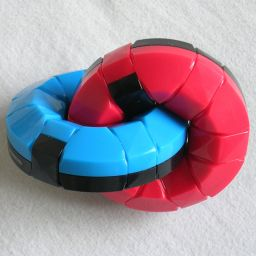# Dual CircleDual Circle consists of two tightly linked rings. Each ring has six sections. If a ring is rotated about its axis, the section on the other ring that is located in the hole of the first ring will be rotated too. The rings are coloured so that the orientation of the 12 sections is visible.

This puzzle is produced by Hanayama, and was invented by Bram Cohen and Oskar van Deventer. Bram came up with the idea of how the pieces move and affect each other, but had them arranged in two parallel rows. He called that puzzle Rotabram. Oskar came up with the design using two linked rings.

If your browser supports JavaScript, then you can play Dual Circle by clicking the link below:

## The number of positions:

There are 12 rotating ring sections, each with 6 possible orientations. This gives a maximum of 612 positions. There is however a twist 'parity' restriction, so only 611 = 362,797,056 positions are possible.

The twist restriction is rather complicated, and not at all obvious. Click the link below for a full explanation.

I have calculated God's Algorithm for this puzzle. It turns out that it can be solved in at most 17 turns (12.992 on average), or 36 moves (20.678 on average) if every sixth of a rotation counts as a move.

Click to view full table.
Ring TurnsSixth Turns
DepthPositions
01
110
250
3250
41,249
56,216
630,720
7144,076
8629,890
92,511,744
109,152,378
1128,273,418
1269,711,614
13117,652,183
14105,615,134
1527,693,510
161,372,965
171,648
Total362,797,056
DepthPositions
01
14
212
334
496
5272
6770
72,152
85,898
915,794
1041,726
11108,152
12275,073
13682,370
141,642,690
153,785,796
168,224,011
1716,436,710
1829,405,280
DepthPositions
1945,583,326
2059,591,176
2164,123,266
2255,809,435
2338,978,212
2421,947,610
2510,142,700
263,947,121
271,379,198
28461,742
29149,240
3043,514
3110,916
322,332
33382
3440
354
361
Total362,797,056

The single antipodal position at 36 sixth turns is the position with all pieces twisted 180 degrees.

## Solution:

Phase 1: Solve the red ring sections

1. Rotate the red ring to bring any unsolved section into the middle of the blue ring.
2. Rotate the blue ring until that red ring section is solved.
3. Repeat steps a-b until the red ring sections are all solved.

Phase 2: Solve the blue ring sections

1. Rotate the red ring so as to solve the blue section currently inside the red ring.
2. Choose a blue ring section that you want to solve.
3. Rotate blue ring to bring the chosen section into the centre of the red ring.
4. Rotate the red ring one step.
5. Rotate the blue ring back to its original position.
6. Rotate the red ring back one step to its original position.
7. Repeat steps c-f until the chosen blue section is solved. Note that this will have disturbed one red ring section.
8. Repeat steps b-f for each unsolved blue section (except for the blue section inside the red ring). Note that in steps d and f you must twist in the same direction as the previous times, so that it is always the same red section that gets disturbed. Once all the blue sections are solved, that disturbed red section should be automatically correct.
9. Rotate the blue ring so as to solve the red section currently inside the blue ring.

## Useful sequences

Phase 2 in the above solution can take quite a number of moves. It may therefore be quite useful to have some further move sequences in your arsenal to speed things up. Below is a complete set of move sequences that only affect one or two pieces in the blue ring. Once you have solved the red ring, you will need to apply no more than four of these sequences to solve the blue ring.

Mentally label the 6 sections of the blue ring with the letters A to F, where A is the one that lies inside the red ring. A letter followed by a + or a - indicates that you have to turn that section one way or the other by one click. Two or three plusses or minuses obviously means it has to move two or three clicks.

EffectMove sequenceEffectMove sequenceEffectMove sequenceEffectMove sequence
B++, C++ B-- C++ B-- B--, C-- B++ C-- B++
B++, C- B+ C- B+ B--, C+ B- C+ B- E-, F++ F+ E- F+ E+, F-- F- E+ F-
B+++, D+ B- C- D+ C+ B-- B+++, D- B+ C+ D- C- B++ D+, F+++F- E- D+ E+ F-- D-, F+++F+ E+ D- E- F++
B+++, D+++B+++ C+++ D+++ C+++ D+++, F+++F+++ E+++ D+++ E+++
B++, E+ B++ A- F- E+ F+ A+ B--, E- B-- A+ F+ E- F- A- C+, F++ F++ A- B- C+ B+ A+ C-, F-- F-- A+ B+ C- B- A-
B++, E-- B+ E- B+ E- B--, E++ B- E+ B- E+ C--, F++ F+ C- F+ C- C++, F-- F- C+ F- C+
B+, F+ F+ A- B+ A+ B-, F- F- A+ B- A-
B++, F++ F++ A-- B++ A++ B--, F-- F-- A++ B-- A--
B+++, F+++F+++ A--- B+++ A+++
C+++ B+++ C+++ B+++ E+++ F+++ E+++ F+++
C+++, D++ C- D+ C- D+ C- C+++, D-- C+ D- C+ D- C+ D++, E+++E- D+ E- D+ E- D--, E+++E+ D- E+ D- E+
C+, E+ E+ A- C+ A+ C-, E- E- A+ C- A-
C-, E++ E+ C- E+ C+, E-- E- C+ E- C++, E- C+ E- C+ C--, E+ C- E+ C-
C++, E++ E-- C++ E-- C--, E-- C++ E-- C++
C+++, E+++C+++ D+++ E+++ D+++
D++ D+ A- D+ A+ D-- D- A+ D- A-